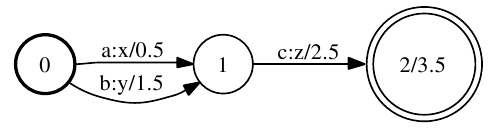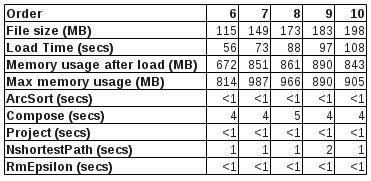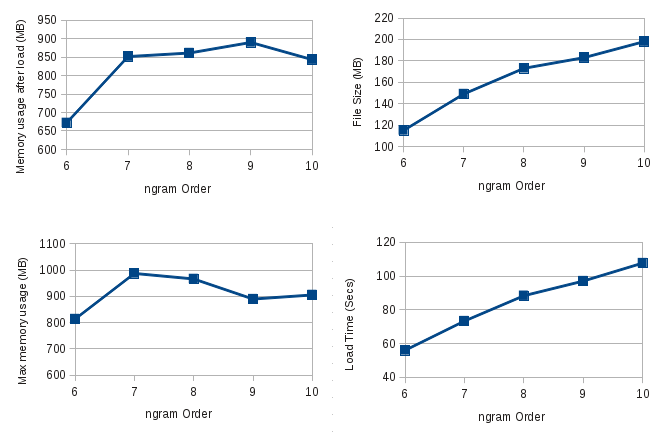(author: John Salatas)

Foreword

This article, the fourth in a series regarding porting openFST to java, describes the latest version of the java fst library, which contains all the required code for porting phonetisaurus g2p decoder  to java and eventually integrate it with sphinx4.

1. Basic Usage

Creating as simple wfst like the one in in figure 1 below is a straightforward procedure

[caption id="attachment_339" align="aligncenter" width="497" caption="Figure 1: Example weighted finite-state transducer"][/caption]

``` // Create a new Semiring TropicalSemiring ts = new TropicalSemiring(); // Create the wfst Fst wfst = new Fst(ts); // Create input and output symbol tables // and assign these to the wfst Mapper isyms = new Mapper(); isyms.put(0, "a"); isyms.put(1, "b"); isyms.put(2, "c"); wfst.setIsyms(isyms);```

```Mapper osyms = new Mapper(); osyms.put(0, "x"); osyms.put(1, "y"); osyms.put(2, "z"); wfst.setIsyms(osyms);```

```// Create state 0 State state = new State(ts.zero()); // Add it to the wfst wfst.addState(state); // Set it as the start state wfst.setStart(s.getId()); // Add arcs to state 0 state.addArc(new Arc(0, 0, 0.5, "1")); state.addArc(new Arc(1, 1, 1.5, "1"));```

``` // Create state 1 state = new State(ts.zero()); // Add it to the wfst wfst.addState(state); // Add arcs to state 1 state.addArc(new Arc(2, 2, 2.5, "2")); ```

``` // Create (final) state 2 state = new State(new Weight(3.5)); // Add it to the wfst wfst.addState(state); ```

Then this wfst can be serialized as binary java fst
``` try { wfst.saveModel("path/to/filename"); } catch (IOException e) { e.printStackTrace(); } ```

or exported to openFst text format

`Convert.export(wfst, wfst.getSemiring(), "path/to/exported");`

Finally it can also be used for several operations as described in the following section.

2. Fst Operations

As already mentioned, priority was given to operations that are required for the porting of phonetisaurus' g2p decoder to java. All operations described are defined in their own class having the same name with the operation under the edu.cmu.sphinx.fst.operations package.

2.1. ArcSort

This operation sorts the arcs in an FST per state. It is defined as follows:
`public static void apply(Fst fst, Comparator cmp)`

The Comparator cmp can be either ILabelCompare or OlabelCompare which short the arcs according to their input or output labels accordingly.

2.2. Compose

This operation computes the composition of two transducers . It is defined as follows:
`public static Fst get(Fst fst1, Fst fst2, Semiring semiring)`

2.3. Connect

This operation removes all states that are not contained on a path leading from the initial state to a final state. It is defined as follows:
`public static void apply(Fst fst)`

2.4. Determinize

This operation determinizes a weighted transducer. The result will be an equivalent FST that has the property that no state has two transitions with the same input label. For this algorithm, epsilon transitions are treated as regular symbols . It is defined as follows:
`public static Fst get(Fst fst)`

2.5. ExtendFinal

This operations extends a wfst by adding a new final state with weight 0 and adding epsilon transitions from the existing final states to the new one, with weights equal to the existing state's final weight. It is defined as follows:
`public static void apply(Fst fst)`

Furthermore there is also a procedure to undo this change, which is defined as:
`public static void undo(Fst fst)`

2.6. NShortestPaths

This operation produces an FST containing the n -shortest paths in the input FST , . It is defined as follows:
`public static Fst get(Fst fst, int n)`

where n is the number of the best paths to return.

2.7. Project

This operation projects an FST onto its domain or range by either copying each arc's input label to its output label or vice versa. It is defined as follows:
`public static void apply(Fst fst, ProjectType pType)`

where pType is an enumeration talking values either INPUT or OUTPUT which project the input labels to the output or the output to the input accordingly.

2.8. Reverse

This operation reverses an FST. Internally it first extends the input to a single final state and this extension is undone before exiting leaving the input fst unchanged. It is defined as follows:
`public static Fst get(Fst fst)`

2.9. RmEpsilon

This operation removes epsilon-transitions (when both the input and output label are an epsilon) from a transducer. The result will be an equivalent FST that has no such epsilon transitions . It is defined as follows:
`public static Fst get(Fst fst)`

3. Performance in g2p decoding

The performance of the fst java library, was evaluated in g2p decoding by porting the phonetisaurus' g2p decoder to java (available at ). N-gram fst models were created (for n=6,7,8,9 and 10) and then loaded in the decoder in order to phoneticize 5 different words. The loading time for each model and also the time of various operations taking place during the decoding, were measured and their average are summarized in the table below. The table also shows the memory used by the java VM after loading the model (this refers more or less to the memory needed by the model) and also the maximum amount of memory utilized during the g2p decoding. All tests were performed on an Intel Core Duo CPU running openSuSE 12.1 x64.

[caption id="attachment_570" align="aligncenter" width="371" caption="Table 1: Performance tests of java g2p decoder"][/caption]

The graphs below visualize the first four rows of the above table.

[caption id="attachment_571" align="aligncenter" width="528" caption="Figure 2: Performance graphs for the java g2p decoder"][/caption]

4. Conclusion – Future work

Studying the performance table in the previous section it is clear that the critical procedure in the decoding process is the model loading (deserialization) which usually take more than a minute to complete. Although this is an issue that needs to be fixed, a quick workaround is to load it in advance and keep a copy of it in memory for providing pronunciations for all required words. This of course comes with additional requirements for memory which are more or less equal to the amount shown in 3rd in the previous section's table (row “Memory usage after load”).

Next step is of course to evaluate the g2p decoder's ability to provide correct pronunciations for words and compare it with the original pronunciations produced by phonetisaurus. Having a same quality java g2p decoder will first of confirm the correctness of the java fst library code and enable us to continue with its integration with CMUSphinx 4.

References
 "Phonetisaurus: A WFST-driven Phoneticizer – Framework Review".
 M. Mohri, "Weighted automata algorithms", Handbook of Weighted Automata. Springer, pp. 213-250, 2009.
 M. Mohri, "Finite-State Transducers in Language and Speech Processing", Computational Linguistics, 23:2, 1997.
 M. Mohri, "Semiring Framework and Algorithms for Shortest-Distance Problems", Journal of Automata, Languages and Combinatorics, 7(3), pp. 321-350, 2002.
 M. Mohri, M. Riley, "An Efficient Algorithm for the n-best-strings problem", Proceedings of the International Conference on Spoken Language Processing 2002 (ICSLP '02).
 G2P java decoder SVN repository